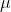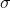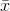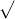##### Question

In: Statistics and Probability

# A population of values has a normal distribution with μ = 49.1 and σ = 29.4...

A population of values has a normal distribution with μ = 49.1 and σ = 29.4 . You intend to draw a random sample of size n = 35 . Find P4, which is the mean separating the bottom 4% means from the top 96% means. P4 (for sample means) =

## Solutions

##### Expert Solution

Solution

Given that,

mean == 49.1

standard deviation == 29.4

n = 35== 49.1=/n = 29.4 /35 = 4.97

Using standard normal table,

P(Z < z) = 4%

= P(Z < z) = 0.04

= P(Z < -1.751) = 0.04

z = -1.751

Using z-score formula= z *+= -1.751 * 4.97 + 49.1= 40.4

## Related Solutions

##### A population of values has a normal distribution with μ = 141.1 and σ = 21.9...
A population of values has a normal distribution with μ = 141.1 and σ = 21.9 . You intend to draw a random sample of size n = 144 . Find P35, which is the score separating the bottom 35% scores from the top 65% scores. P35 (for single values) = Find P35, which is the mean separating the bottom 35% means from the top 65% means. P35 (for sample means) = Enter your answers as numbers accurate to 1...
##### A population of values has a normal distribution with μ = 231.5 and σ = 53.2...
A population of values has a normal distribution with μ = 231.5 and σ = 53.2 . If a random sample of size n = 19 is selected , Find the probability that a single randomly selected value is greater than 241.3. Round your answer to four decimals. P(X > 241.3) = Find the probability that a sample of size n=19n=19 is randomly selected with a mean greater than 241.3. Round your answer to four decimals. P(M > 241.3) =
##### A population of values has a normal distribution with μ = 247.3 and σ = 5.7....
A population of values has a normal distribution with μ = 247.3 and σ = 5.7. You intend to draw a random sample of size n = 12. Find P72, which is the mean separating the bottom 72% means from the top 28% means. P72 (for sample means) = Enter your answers as numbers accurate to 1 decimal place. Answers obtained using exact z-scores or z-scores rounded to 3 decimal places are accepted.
##### A population of values has a normal distribution with μ = 240.5 and σ = 70.2...
A population of values has a normal distribution with μ = 240.5 and σ = 70.2 . You intend to draw a random sample of size n = 131 . Find the probability that a single randomly selected value is between 246 and 249.7. P(246 < X < 249.7) Find the probability that a sample of size n = 131 is randomly selected with a mean between 246 and 249.7. P(246 < M < 249.7)
##### A population of values has a normal distribution with μ = 107.5 and σ = 9.7...
A population of values has a normal distribution with μ = 107.5 and σ = 9.7 . A random sample of size n = 133 is drawn. a.) Find the probability that a single randomly selected value is between 106.4 and 110. Round your answer to four decimal places. P ( 106.4 < X < 110 ) = b.) Find the probability that a sample of size n = 133 is randomly selected with a mean between 106.4 and 110....
##### A population of values has a normal distribution with μ = 180.2 and σ = 17.4...
A population of values has a normal distribution with μ = 180.2 and σ = 17.4 . You intend to draw a random sample of size n = 235 . Find the probability that a single randomly selected value is between 177.2 and 177.7. P(177.2 < X < 177.7) = Find the probability that a sample of size n = 235 is randomly selected with a mean between 177.2 and 177.7. P(177.2 < M < 177.7) =
##### 1) A population of values has a normal distribution with μ = 97.3 and σ =...
1) A population of values has a normal distribution with μ = 97.3 and σ = 21.5 . You intend to draw a random sample of size n = 42 . A) Find the probability that a single randomly selected value is greater than 107.3. P(X > 107.3) = Round to 4 decimal places. B) Find the probability that the sample mean is greater than 107.3. P( ¯¯¯ X > 107.3) = Round to 4 decimal places. Answers obtained using...
##### A population of values has a normal distribution with μ = 127.5 and σ = 96.9...
A population of values has a normal distribution with μ = 127.5 and σ = 96.9 . You intend to draw a random sample of size n = 150 . Find the probability that a single randomly selected value is greater than 114.8. P(x > 114.8) = Find the probability that a sample of size n = 150 is randomly selected with a mean greater than 114.8. P( ¯ x > 114.8) = Enter your answers as numbers accurate to...
##### A population of values has a normal distribution with μ = 109.2 and σ = 65.6...
A population of values has a normal distribution with μ = 109.2 and σ = 65.6 . You intend to draw a random sample of size n = 90 . Find the probability that a sample of size n = 90 is randomly selected with a mean greater than 96.1. P( ¯ x > 96.1) = Enter your answers as numbers accurate to 4 decimal places. Answers obtained using exact z-scores or z-scores rounded to 3 decimal places are accepted....
##### A population of values has a normal distribution with μ = 128.5 and σ= 26.6 You...
A population of values has a normal distribution with μ = 128.5 and σ= 26.6 You intend to draw a random sample of size n=58 Find the probability that a single randomly selected value is greater than 123.3. P(X > 123.3) = Find the probability that a sample of size n= 58 is randomly selected with a mean greater than 123.3. P(M > 123.3) =